# Motivation

23. Nov 2011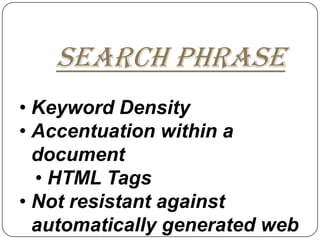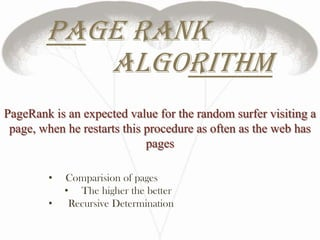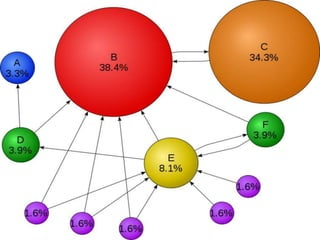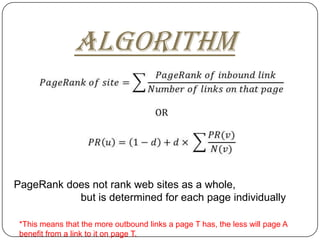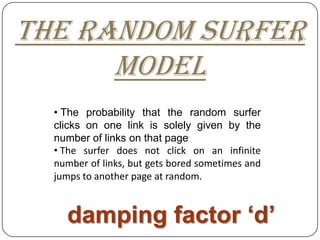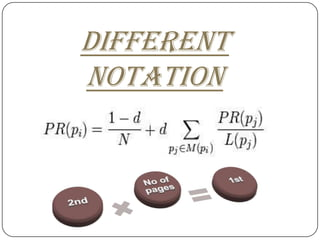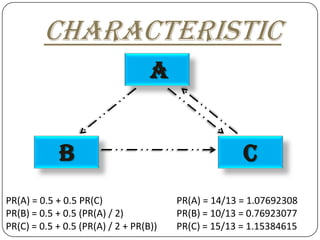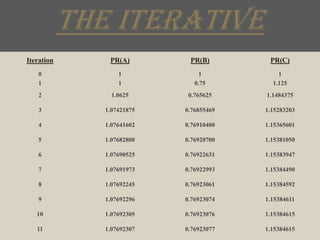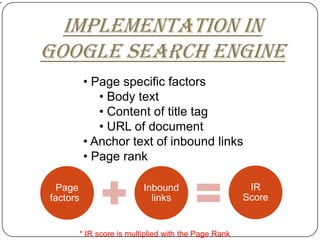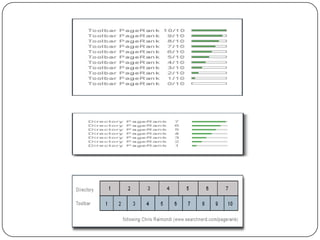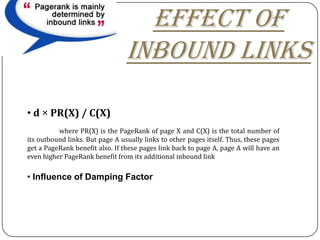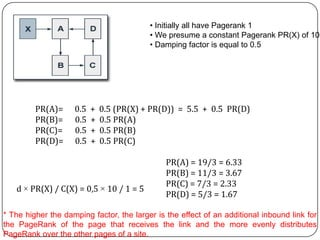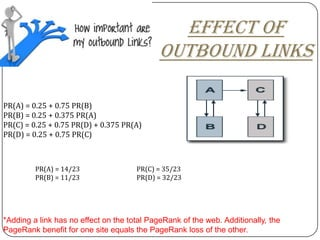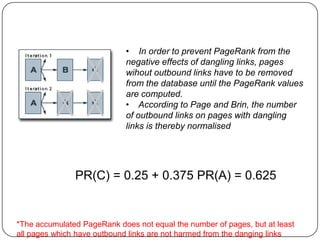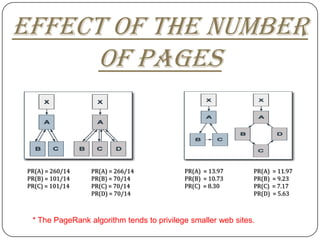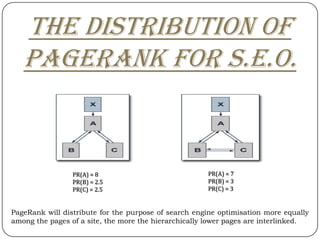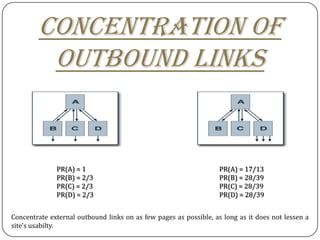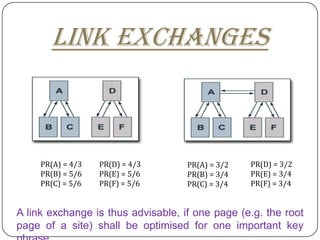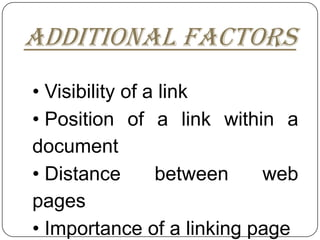1 von 28

### Motivation

• 1. Motivation 1. Link algorithm(SALSA) 2. Page Rank Algorithm
• 2. SEARCH PHRASE • Keyword Density • Accentuation within a document • HTML Tags • Not resistant against automatically generated web
• 3. Link popularity algorithm • Inbound Links. • Decieve Search Engines • Creating masses of inbound links
• 6. Page rank algorithm PageRank is an expected value for the random surfer visiting a page, when he restarts this procedure as often as the web has pages • Comparision of pages • The higher the better • Recursive Determination
• 8. introduction Sergey Brin Larry Page
• 9. algorithm PageRank does not rank web sites as a whole, but is determined for each page individually *This means that the more outbound links a page T has, the less will page A benefit from a link to it on page T.
• 10. The random surfer model • The probability that the random surfer clicks on one link is solely given by the number of links on that page • The surfer does not click on an infinite number of links, but gets bored sometimes and jumps to another page at random. damping factor ‘d’
• 11. Different notation
• 12. characteristic A b c PR(A) = 0.5 + 0.5 PR(C) PR(A) = 14/13 = 1.07692308 PR(B) = 0.5 + 0.5 (PR(A) / 2) PR(B) = 10/13 = 0.76923077 PR(C) = 0.5 + 0.5 (PR(A) / 2 + PR(B)) PR(C) = 15/13 = 1.15384615
• 13. The iterative Iteration PR(A) PR(B) PR(C) 0 1 1 1 1 1 0.75 1.125 2 1.0625 0.765625 1.1484375 3 1.07421875 0.76855469 1.15283203 4 1.07641602 0.76910400 1.15365601 5 1.07682800 0.76920700 1.15381050 6 1.07690525 0.76922631 1.15383947 7 1.07691973 0.76922993 1.15384490 8 1.07692245 0.76923061 1.15384592 9 1.07692296 0.76923074 1.15384611 10 1.07692305 0.76923076 1.15384615 11 1.07692307 0.76923077 1.15384615
• 14. Implementation in google search engine • Page specific factors • Body text • Content of title tag • URL of document • Anchor text of inbound links • Page rank Page Inbound IR factors links Score * IR score is multiplied with the Page Rank
• 16. Effect of inbound links • d × PR(X) / C(X) where PR(X) is the PageRank of page X and C(X) is the total number of its outbound links. But page A usually links to other pages itself. Thus, these pages get a PageRank benefit also. If these pages link back to page A, page A will have an even higher PageRank benefit from its additional inbound link • Influence of Damping Factor
• 17. • Initially all have Pagerank 1 • We presume a constant Pagerank PR(X) of 10 • Damping factor is equal to 0.5 PR(A)= 0.5 + 0.5 (PR(X) + PR(D)) = 5.5 + 0.5 PR(D) PR(B)= 0.5 + 0.5 PR(A) PR(C)= 0.5 + 0.5 PR(B) PR(D)= 0.5 + 0.5 PR(C) PR(A) = 19/3 = 6.33 PR(B) = 11/3 = 3.67 PR(C) = 7/3 = 2.33 d × PR(X) / C(X) = 0,5 × 10 / 1 = 5 PR(D) = 5/3 = 1.67 * The higher the damping factor, the larger is the effect of an additional inbound link for the PageRank of the page that receives the link and the more evenly distributes PageRank over the other pages of a site.
• 18. Effect of outbound links PR(A) = 0.25 + 0.75 PR(B) PR(B) = 0.25 + 0.375 PR(A) PR(C) = 0.25 + 0.75 PR(D) + 0.375 PR(A) PR(D) = 0.25 + 0.75 PR(C) PR(A) = 14/23 PR(C) = 35/23 PR(B) = 11/23 PR(D) = 32/23 *Adding a link has no effect on the total PageRank of the web. Additionally, the PageRank benefit for one site equals the PageRank loss of the other.
• 19. Dangling links PR(A) = 0.25 + 0.75 PR(B) PR(B) = 0.25 + 0.375 PR(A) PR(C) = 0.25 + 0.375 PR(A) PR(A) = 14/23 PR(B) = 11/23 PR(C) = 11/23 Dangling links could have major impacts on PageRank.
• 20. • In order to prevent PageRank from the negative effects of dangling links, pages wihout outbound links have to be removed from the database until the PageRank values are computed. • According to Page and Brin, the number of outbound links on pages with dangling links is thereby normalised PR(C) = 0.25 + 0.375 PR(A) = 0.625 *The accumulated PageRank does not equal the number of pages, but at least all pages which have outbound links are not harmed from the danging links
• 21. Effect of the number of pages PR(A) = 260/14 PR(A) = 266/14 PR(A) = 13.97 PR(A) = 11.97 PR(B) = 101/14 PR(B) = 70/14 PR(B) = 10.73 PR(B) = 9.23 PR(C) = 101/14 PR(C) = 70/14 PR(C) = 8.30 PR(C) = 7.17 PR(D) = 70/14 PR(D) = 5.63 * The PageRank algorithm tends to privilege smaller web sites.
• 22. The distribution of pagerank for s.e.o. PR(A) = 8 PR(A) = 7 PR(B) = 2.5 PR(B) = 3 PR(C) = 2.5 PR(C) = 3 PageRank will distribute for the purpose of search engine optimisation more equally among the pages of a site, the more the hierarchically lower pages are interlinked.
• 23. Concentration of outbound links PR(A) = 1 PR(A) = 17/13 PR(B) = 2/3 PR(B) = 28/39 PR(C) = 2/3 PR(C) = 28/39 PR(D) = 2/3 PR(D) = 28/39 Concentrate external outbound links on as few pages as possible, as long as it does not lessen a site's usabilty.
• 24. Link exchanges PR(A) = 4/3 PR(D) = 4/3 PR(A) = 3/2 PR(D) = 3/2 PR(B) = 5/6 PR(E) = 5/6 PR(B) = 3/4 PR(E) = 3/4 PR(C) = 5/6 PR(F) = 5/6 PR(C) = 3/4 PR(F) = 3/4 A link exchange is thus advisable, if one page (e.g. the root page of a site) shall be optimised for one important key
• 25. Additional factors • Visibility of a link • Position of a link within a document • Distance between web pages • Importance of a linking page
• 26. Bibliography • Wikipedia • Pr.efactory.de • Youtube • Google Images
• 27. questionnaires